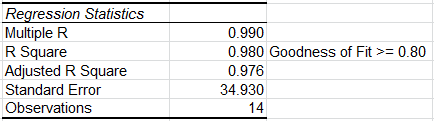# Adjusted R Squared in Excel

## You Don't Have to be a Statistician to understand R Squared and Adjusted R Squared

Regression analysis evaluates the effects of one or more independent variables on a single dependent variable. Regression arrives at an equation to predict performance based on each of the inputs.

The R Squared and Adjusted R Squared values give the goodness of fit. Anything over 0.80 (80%) is considered a good fit.

R Squared can be biased. So, Adjusted R Squared tweaks the R Squared based on degrees of freedom.

### Example of QI Macros ResultsAnalysis: If R Squared is greater than 0.80, as it is in this case, there is a good fit to the data. Some statistics references recommend using the Adjusted R Squared value.

In this example, R Squared of 0.980 means that 98% of the variation can be explained by the independent variables. Notice that the Adjusted R Squared (0.976) is less than R Squared (.980).

### Why Choose QI Macros Statistical Software for Excel?### Easy to Use

• Works Right in Excel
• Interprets p-values for You
• Accurate No-Worry Results
• Free Training Anytime### Proven and Trusted

• 100,000 Users in 80 Countries
• Celebrating 20th Anniversary
• Five Star CNET Rating - Virus Free### Affordable

• Only \$349 USD
Quantity Discounts Available
• No annual fees
• Free Technical Support